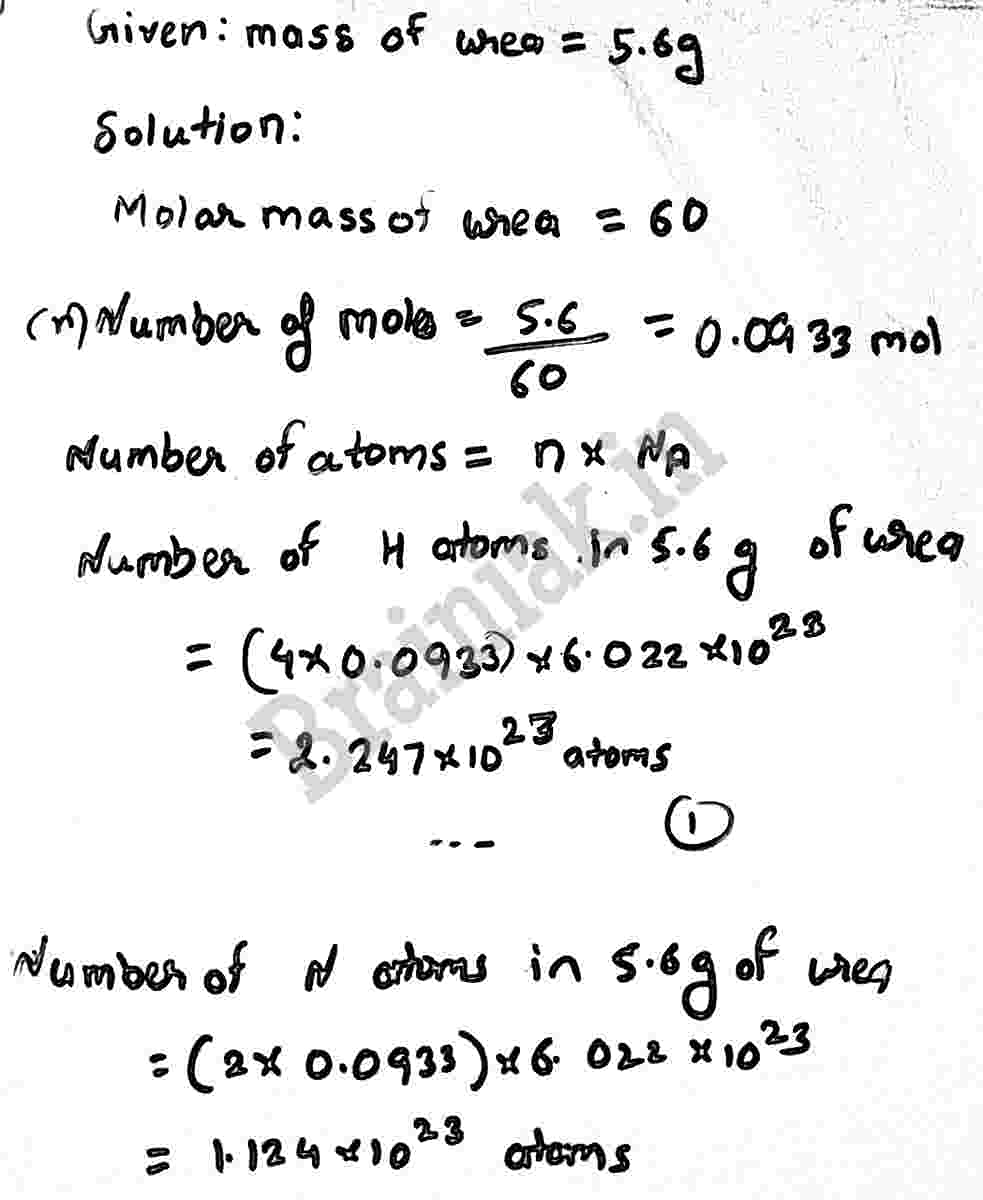# Q. Calculate the number of atoms of hydrogen present in 5.6 g of urea, (NH2 )2 CO

more_vert

4 . solve problems

Q. Calculate the number of atoms of hydrogen present in 5.6 g of urea, (NH2)2CO. Also calculate the number of atoms of N, C and O.

more_vert

verified​​​​​​